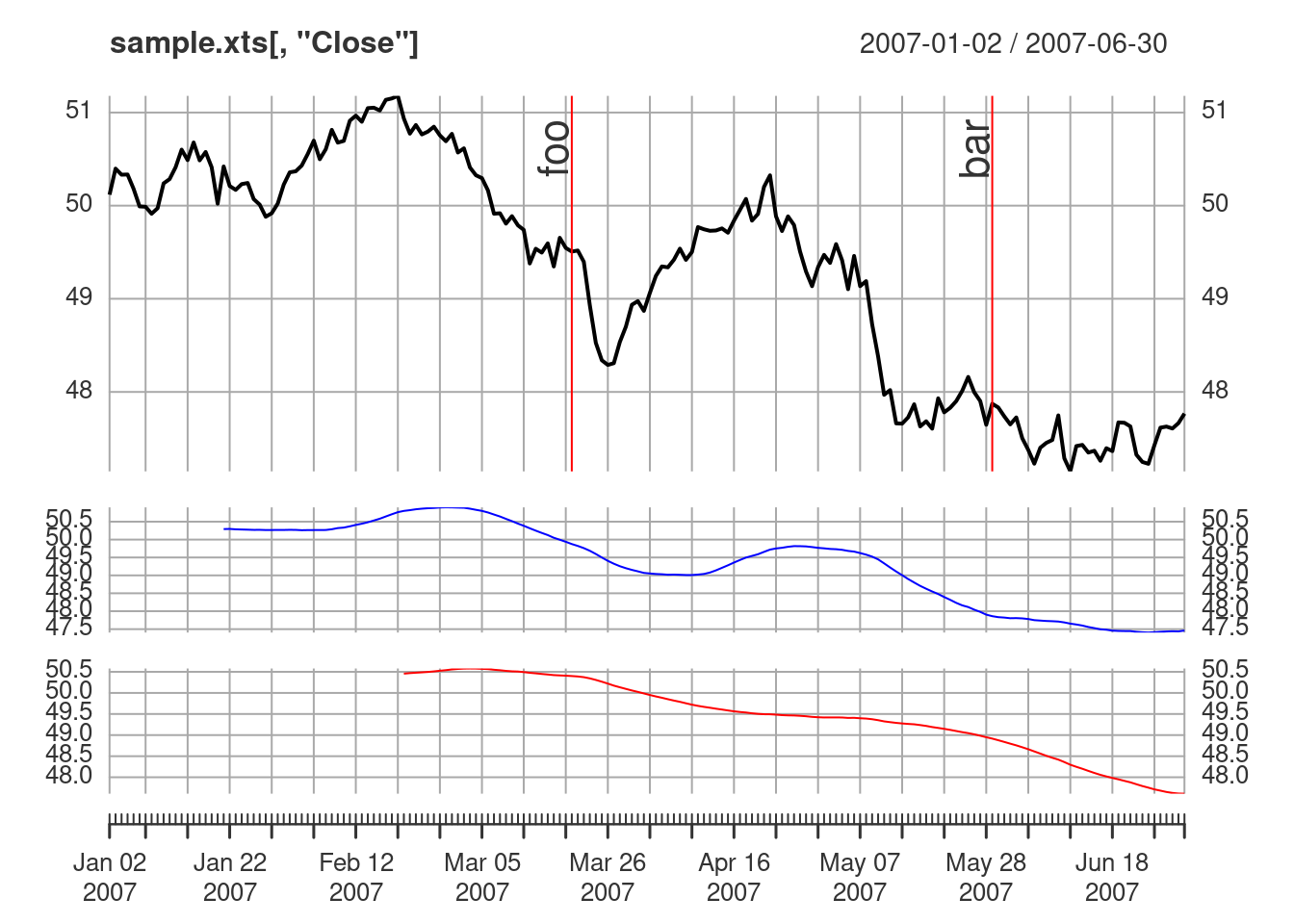### Basics

Load the xts package and some sample data

``````library(xts)

data(sample_matrix)
sample.xts <- as.xts(sample_matrix)``````

Create a basic plot.

``plot(sample.xts[,"Close"])``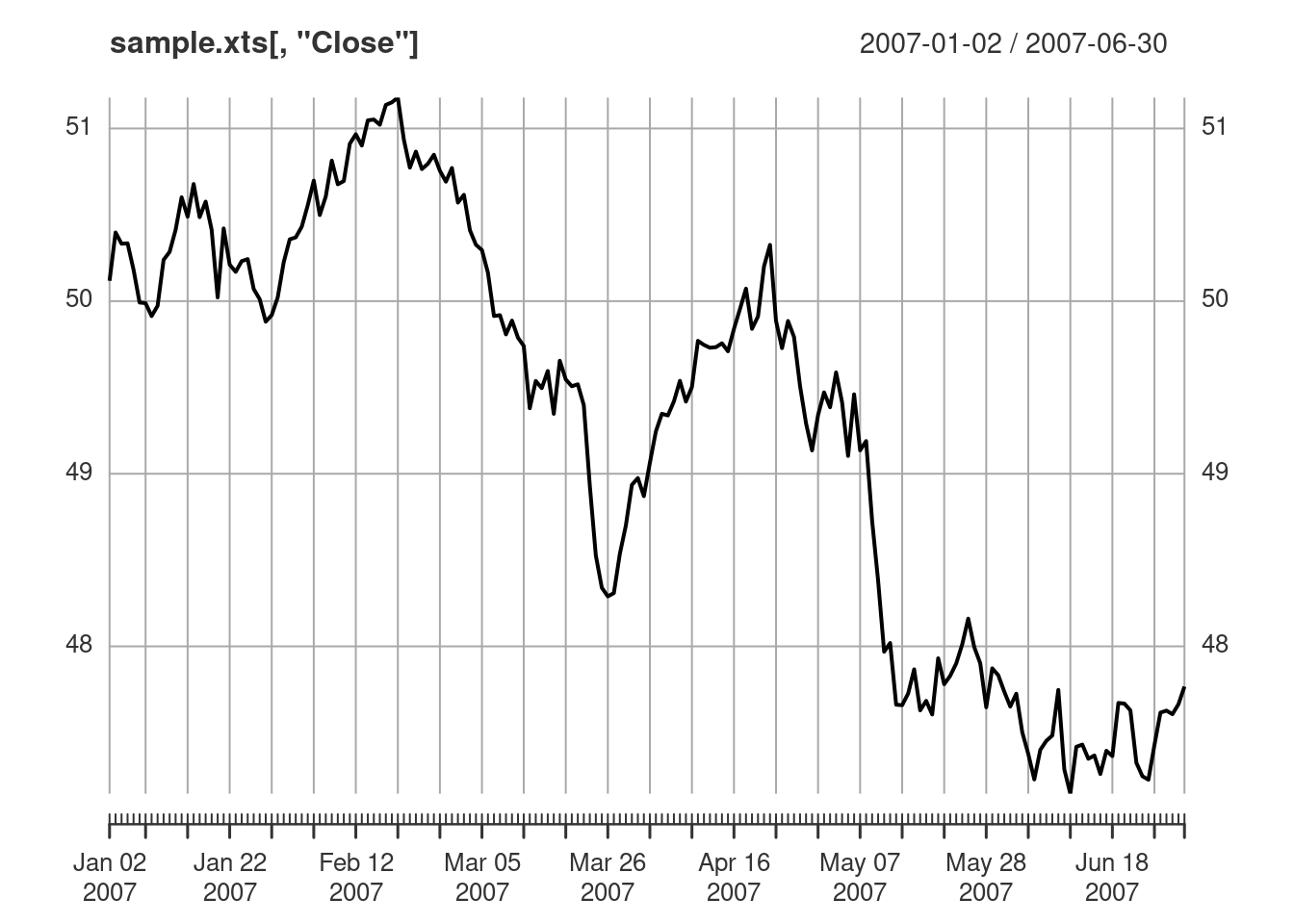Subset to only plot March 2007. The `subset` argument uses xts subsetting described in `?xts`.

``plot(sample.xts[,"Close"], subset="2007-03")``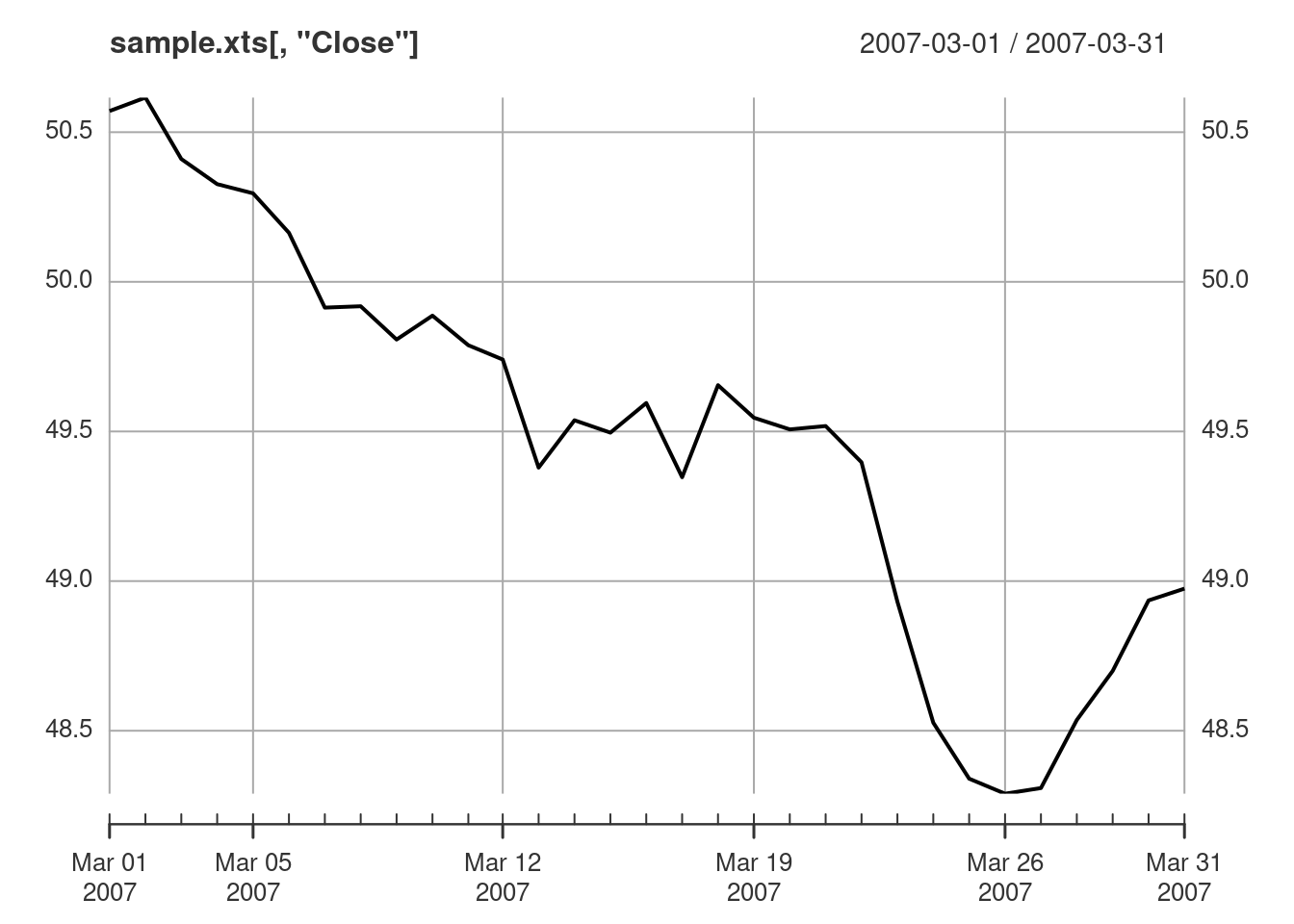Customize the plot by changing the color (`col`), line type (`lty`), and line width (`lwd`). See `?par` for more valid values for `lty` and `lwd`.

``plot(sample.xts[,"Close"], col="blue", lwd=2, lty=2)``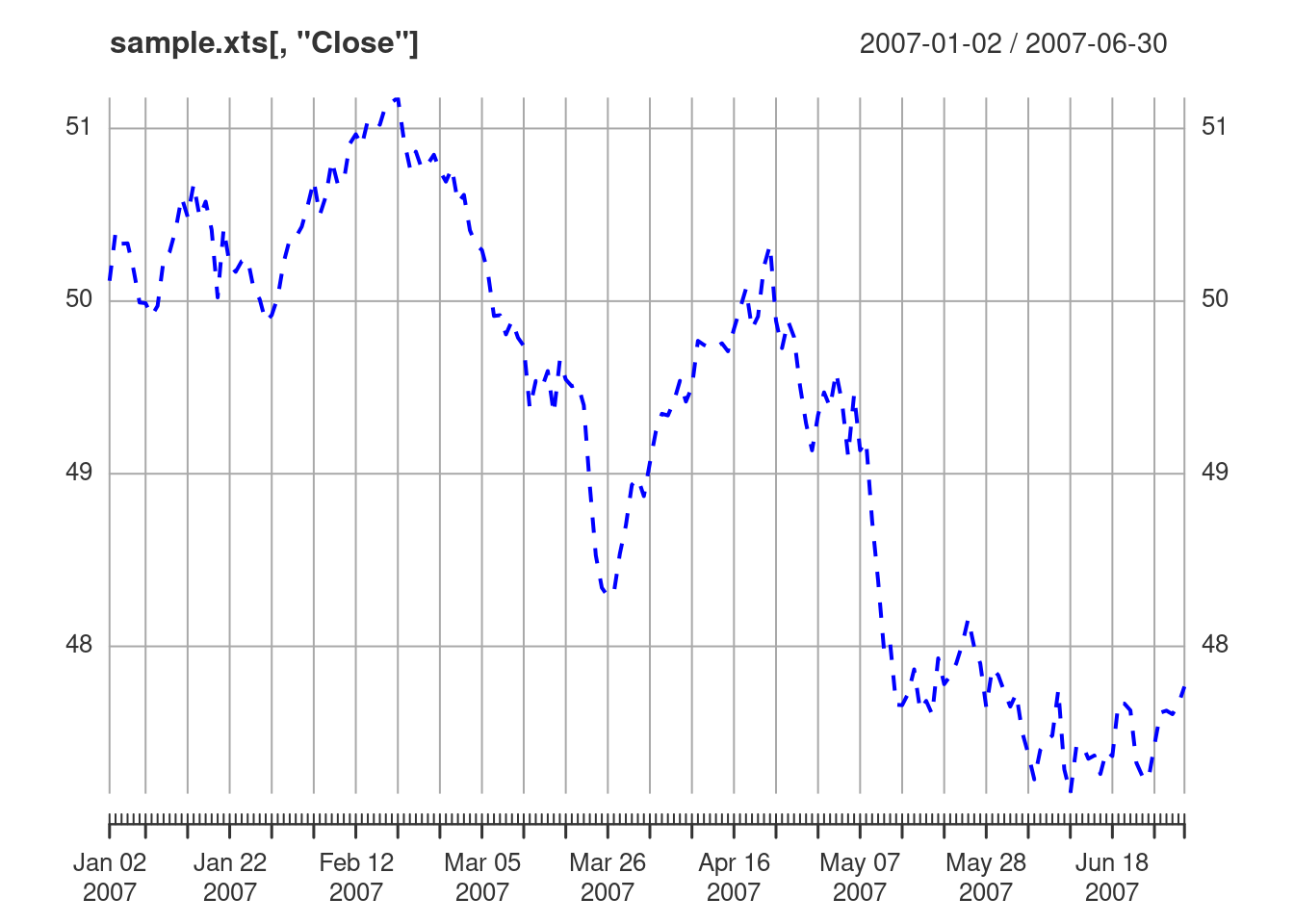### Lines

Here we start with a basic plot, then add moving average lines, and a legend. The default behavior for `lines` is to add to the current panel. The current panel is defined as the panel on which the most recent action was performed. Note that the default behavior for `addLegend` is also to add to the current panel.

``````plot(sample.xts[,"Close"])
lines(TTR::SMA(sample.xts[,"Close"], n = 20), col="blue")
lines(TTR::SMA(sample.xts[,"Close"], n = 50), col="red", lty=2)

# add legend to panel 1
addLegend("topright", legend.names = c("Close", "SMA(20)", "SMA(50)"),
lty=c(1, 1, 2), lwd=c(2, 1, 1),
col=c("black", "blue", "red"))``````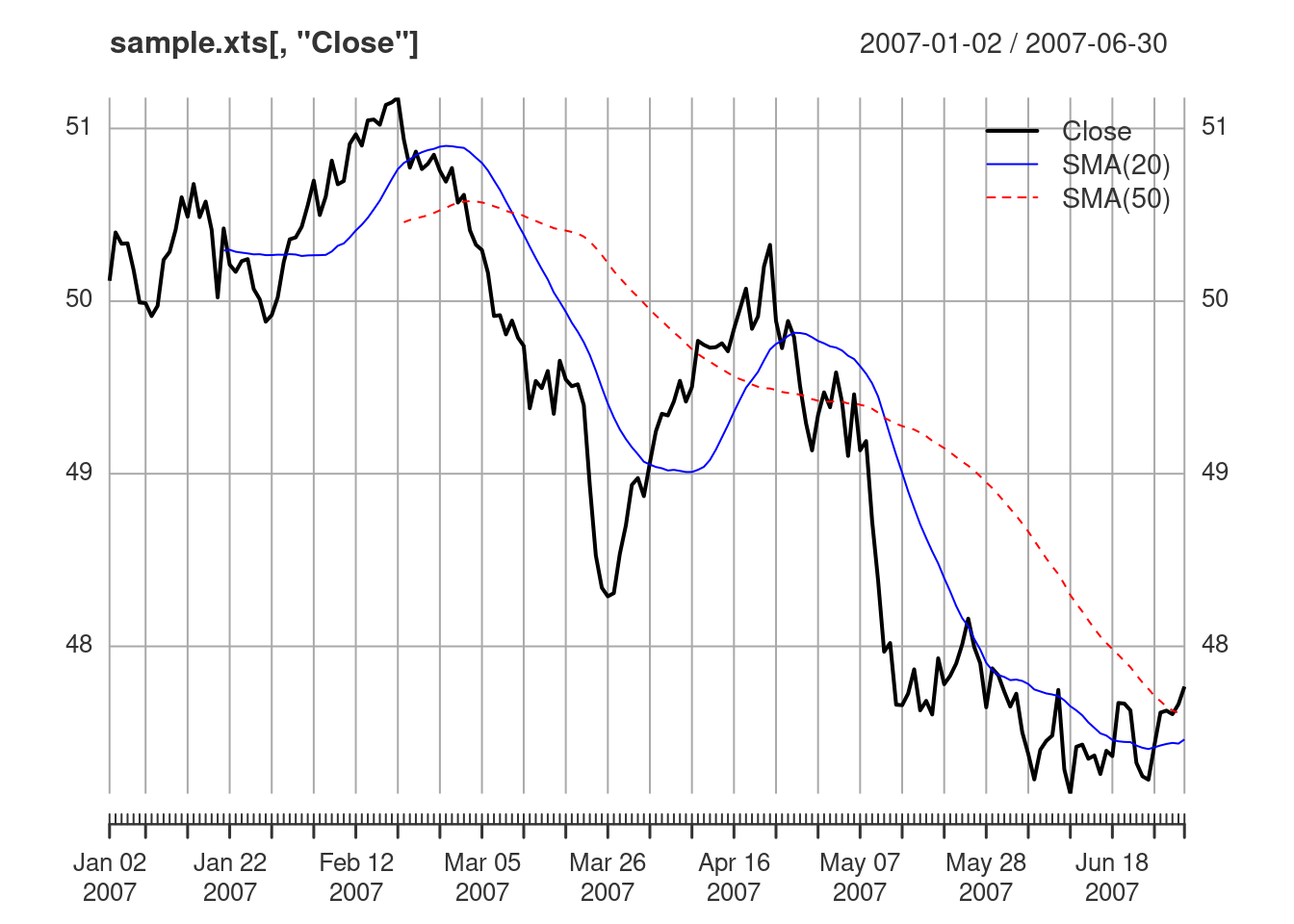A new panel is drawn by specifying `on=NA`. To draw lines on a specific panel, pass an integer corresponding to the panel number to `on`.

``````plot(sample.xts[,"Close"])
lines(TTR::SMA(sample.xts[,"Close"], n = 20), on=NA, col="blue")
lines(TTR::SMA(sample.xts[,"Close"], n = 50), on=NA, col="red")
# draw a line on panel 3
lines(TTR::SMA(sample.xts[,"Close"], n = 60), on=3, col="darkred")
# draw a line on panel 2
lines(TTR::SMA(sample.xts[,"Close"], n = 25), on=2, col="darkblue")
# draw a line on the current panel
lines(TTR::SMA(sample.xts[,"Close"], n = 10), col="green", lty=2)
# draw a line on panel 1
lines(TTR::SMA(sample.xts[,"Close"], n = 100), on=1, col="lightblue")

# add legend to the current panel
lty=c(1, 1), lwd=c(2, 1),
col=c("black", "lightblue"))``````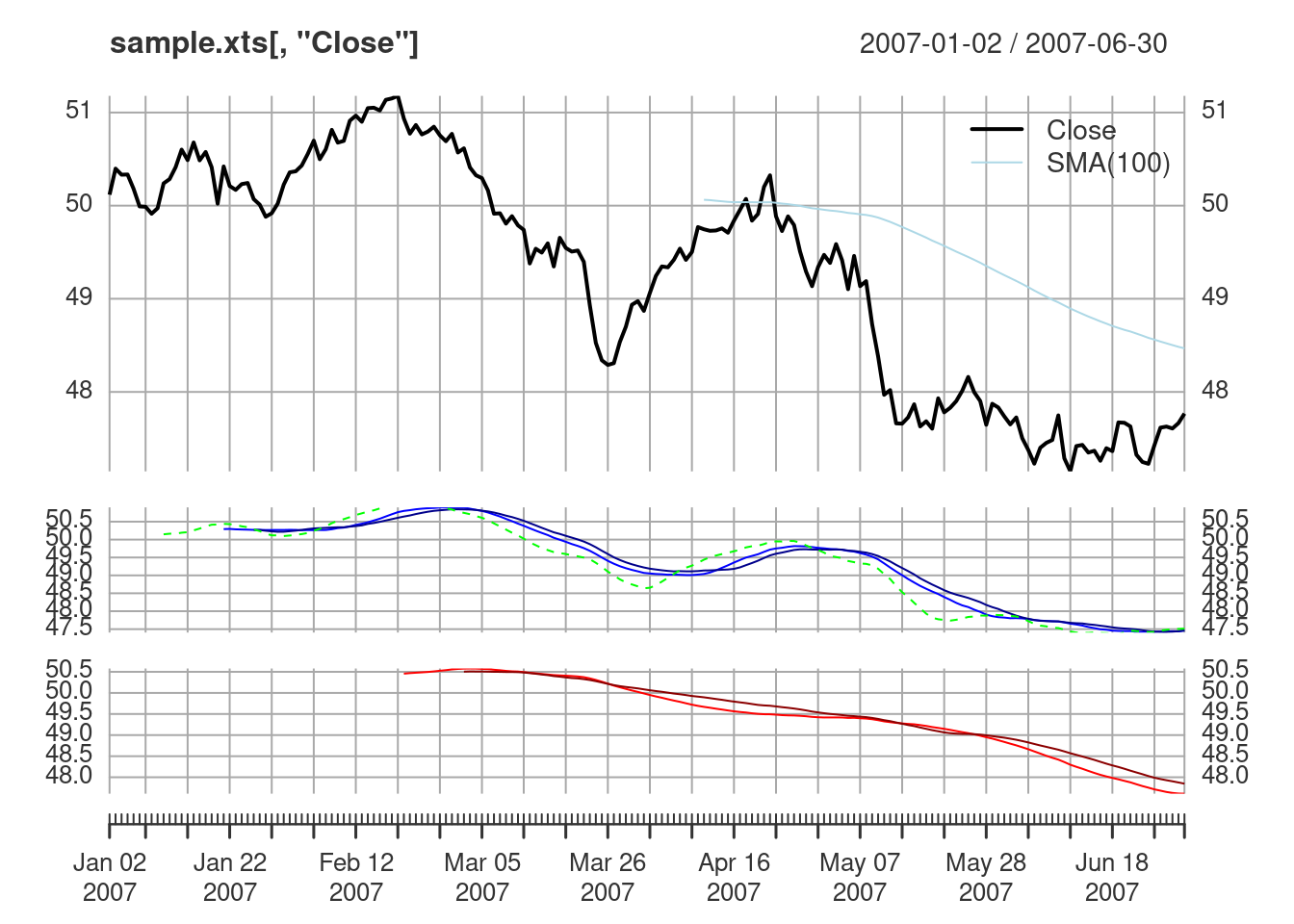### Points

We can also add points to a plot in the same way we added lines. The default behavior for `points` is the same as `lines`, which is to draw the points on on the current panel.

``````plot(sample.xts[,"Close"])
points(TTR::SMA(sample.xts[,"Close"], n = 20), col="blue", pch=2)
points(TTR::SMA(sample.xts[,"Close"], n = 50), col="red", pch=5)

# add legend to panel 1
addLegend("topright", legend.names = c("Close", "SMA(20)", "SMA(50)"),
lty=c(1, NA, NA), pch=c(NA, 2, 5),
col=c("black", "blue", "red"))``````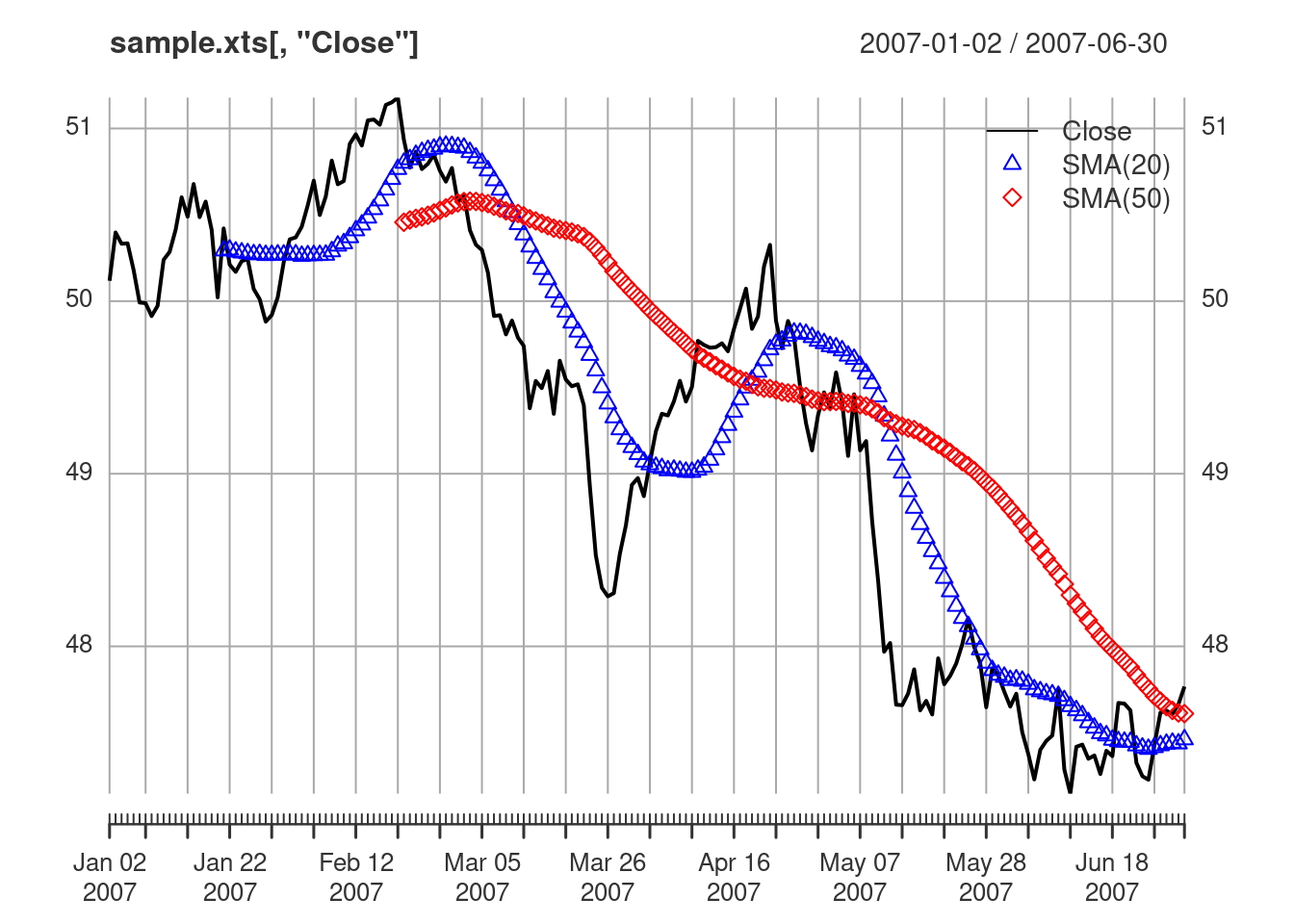### Event Lines

`addEventLines` will draw lines on the current panel just as in `lines`, `points`, and `addLegend`.

``````plot(sample.xts[,4])
events <- xts(c("foo", "bar"), as.Date(c("2007-03-20","2007-05-28")))
addEventLines(events, offset=1.2, pos=2, , srt=90, cex=1.5)
addEventLines(events, offset=1.2, pos=4, , srt=-90, cex=1.5)``````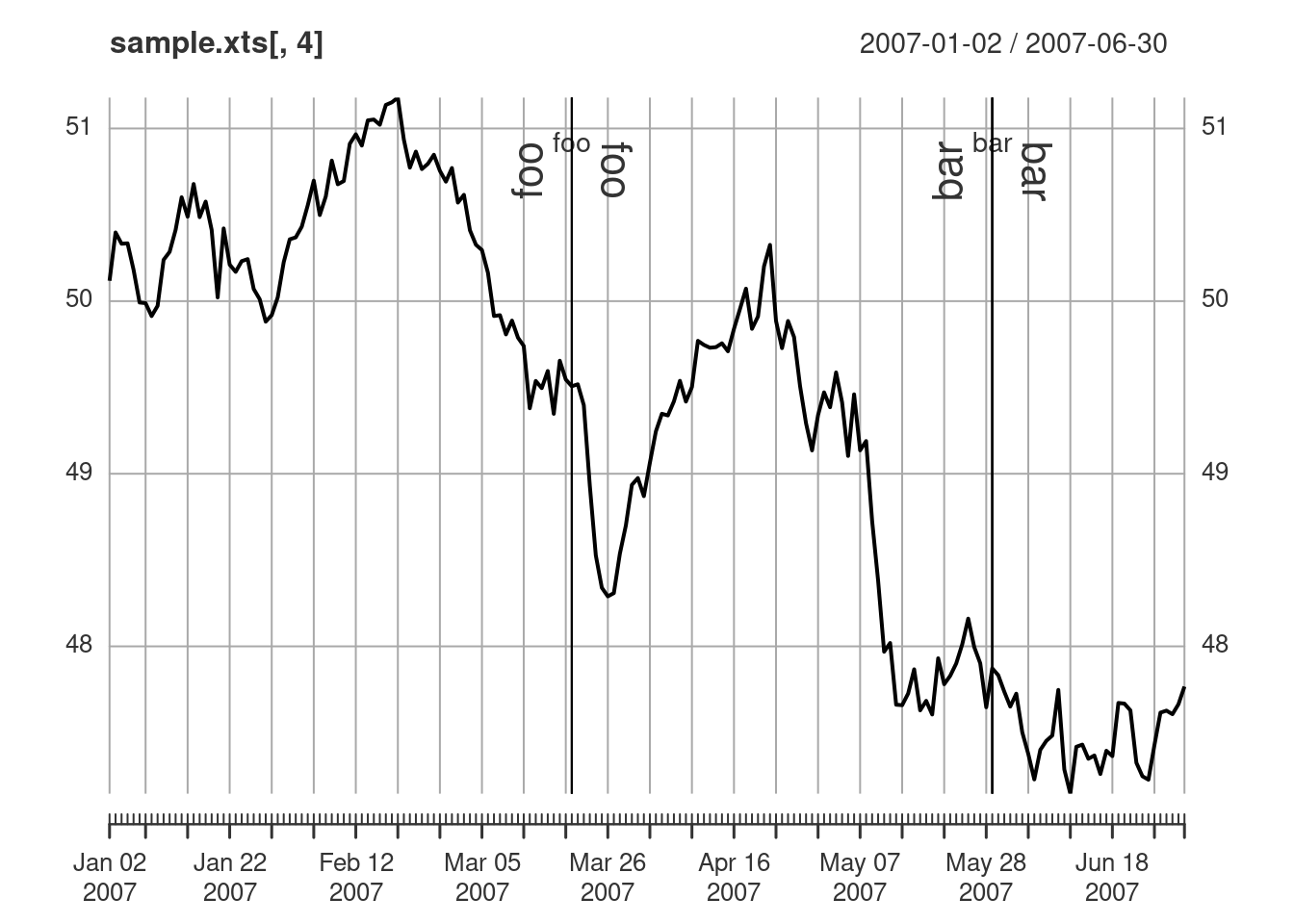Event lines can be drawn on a specific panel by specifying an integer corresponding to the desired panel for `on`. A new panel is drawn if `on=NA`.

``````plot(sample.xts[,"Close"])